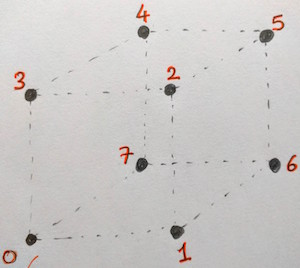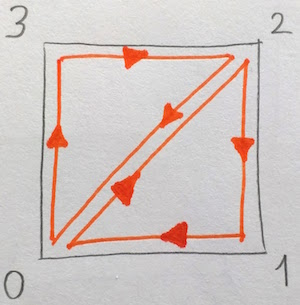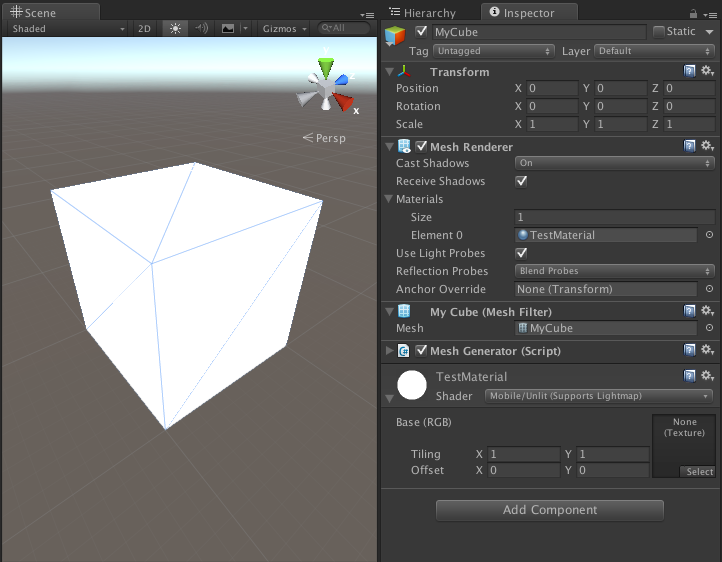Meshes are created from very simple elements, points and lines. The points are called vertices, and a single point is called a vertex. Vertices define points in 3D space. In Unity3D, three connected vertices form a triangle and these triangles define the mesh of objects.

Unity lets us create dynamic meshes with scripts. Creating meshes on the fly (at runtime) allows us to create procedurally generated terrain, for example a voxel terrain like MineCraft uses can be built with dynamic meshes in unity.

We are going to create a cube mesh from script. So lets start by defining the vertices of our cube.Picture above shows 8 vertices numbered through 0 to 7. Vertex index 0 is at position [0, 0, 0] and each edge of the cube has a size of 1 unit. The vertex array for the above cube is as follows:

The next step is to create triangles. Triangles forming the cube are represented by an integer array. Each element of the array is the index of a vertex in the vertices array. For example the picture below is the front face of the cube. It is composed of 2 triangles. The first triangle is created by vertices [0, 2, 1] and the second is created by vertices [0, 3, 2].The order of the vertices in each triangle is called the winding order. Winding order can be used to determine whether the triangle is being seen from the front or the back side. Unity3D uses clockwise winding order for determining front-facing triangles. The cube has 6 faces composed of 12 triangles. Below is the sample code for triangles array. It uses the indices of the vertices previously defined.

Here is the full script. RequireComponent attribute automatically adds required components as dependencies. When you attach this script to a game object in the scene MeshFilter and MeshRenderer components will be automatically added to the game object.

And picture below shows the final cube.Next post shows how to apply a texture to the cube mesh we have just created.